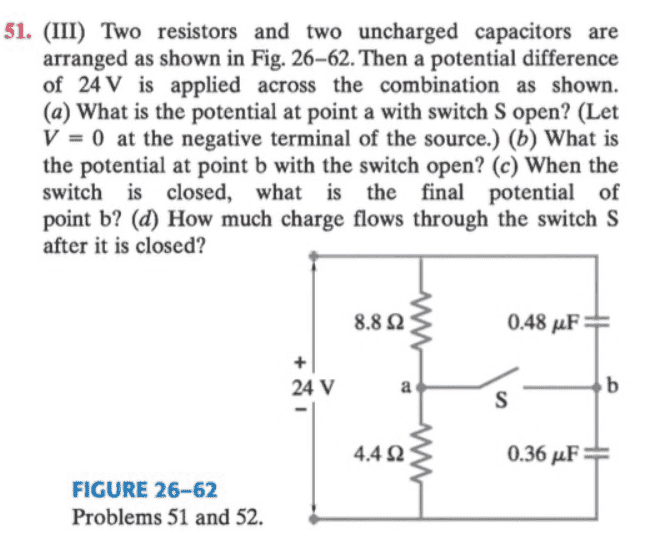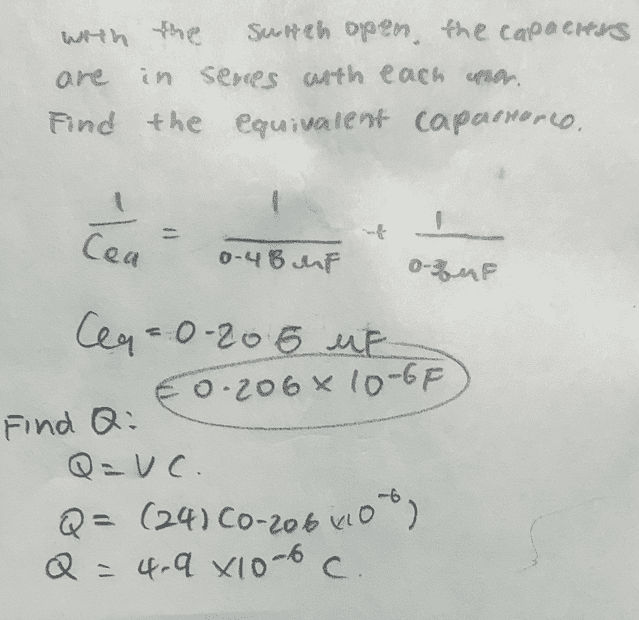# Finding potential at a point in capacitor

• Sunwoo Bae
In summary, the conversation discusses using Kirchhoff's law to solve for the current value and Ohm's law to calculate the voltage at point a. It also presents a question about which resistance and capacitance values to use in calculations.

#### Sunwoo Bae

Homework Statement
shown below
Relevant Equations
Ohm's law, Kirchhoff law,I tried solving the part (a), and got I =1.82 A for the current value using Kirchoff's law.
Next, I want to use Ohm's law to calculate the voltage at point a.
Va = IR
In this equation, will resistance R correspond to 4.4Ω or 8.8Ω?
How do you determine which resistance to use when solving this problem?

As for part B, I was able to calculate Ceq and Q through following works:I am now trying to find the potential at point b with the switch open through equation
Vb = Q/c
Again, which value of capacitance, 0.48 µF or 0.36 µF, and why?

Thank you!

Have you come across the idea of a potential divider? The voltage across either resistors is in proportion to the value of the resistance. The same idea carries across for the capacitors, except the weights are the reciprocals ##1/C##. Can you show why this is true?

Sunwoo Bae said:
Homework Statement:: shown below
Relevant Equations:: Ohm's law, Kirchhoff law,

View attachment 296733

I tried solving the part (a), and got I =1.82 A for the current value using Kirchoff's law.
Next, I want to use Ohm's law to calculate the voltage at point a.
Va = IR
In this equation, will resistance R correspond to 4.4Ω or 8.8Ω?
How do you determine which resistance to use when solving this problem?

As for part B, I was able to calculate Ceq and Q through following works:
View attachment 296735

I am now trying to find the potential at point b with the switch open through equation
Vb = Q/c
Again, which value of capacitance, 0.48 µF or 0.36 µF, and why?

Thank you!
You can use either resistor value.

You can use either capacitor value.

The problem states to use a potential value of 0 V at the negative terminal of the source.
What is the potential value at the positive terminal?